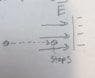# Electron brought to rest by the E-field, potential difference question

In summary, an electron was brought to rest by an electric field and had a potential energy of -0.712V.f

## Homework Statement

An Electron with an initial speed of 500,000m/s is brought to rest by an electric field
a)did the electron move into a region of higher or lower potential? I
b) what was the potential difference that stopped the electron?

ΔV=ΔU/q
ΔU=-W

## The Attempt at a Solution

a) my logic: if the electron is brought to rest that would mean the thing creating the electric field would be negative (since like charges repel each other). So I am imagining something like Electric Field.jpg attached.
Since I know that ΔV=ΔU/q, an electron would have a very high potential energy when near a negative plate (like in my pic). That means the closer it travels in the direction of the charged plate it is gaining electric potential based on the equation ΔV=ΔU/q, since q is not changing.
So I believe the electron traveled from a region of lower electric potential to higher electric potential.
when the problem states "did the electron move into a region of higher or lower potential" they are saying electric potential, correct? just want to clarify

b)
ΔV=ΔU/q
ΔU=-W
W=ΔK=(1/2)mvf2-(1/2)mvi2
combining these equations:
ΔV=-[1/2)mvf2-(1/2)mvi2]/q
the electrons is brought to rest so vf=0
so the equation becomes:
ΔV=(1/2)mvi2/q→(1.1387x10-19)/(-1.602x10-19)=-0.712V
Why do we have to use -e for electron? I know the charge on an electron is -1.602x10^-19, however I remember in the last few chapters when talking about electric fields etc sometimes we didn't have to use the negative value of the electron

#### Attachments

•Electric Field.jpg
24.9 KB · Views: 622
Last edited:
What was the initial kinetic energy of the electron, given its initial velocity? Express your answer in Joules initially, and then in eV. What was the final kinetic energy in eV. What was the decelerating potential...?•... an electron would have a very high potential ...
Electrons don't have electric potential V. They have potential energy U = qV, where, for an electron, q = -1.6×0-19C. I suggest that you figure out the answers to the following three questions
1. Do electric field lines point from a region of high electric potential V to a region of low electric potential V or the other way around?
2. When an electron moving with some initial speed is stopped by an electric field, does it move from a region of low electric potential energy U to a region of high electric potential energy U or the other way around?
3. When an electron moving with some initial speed is stopped by an electric field, does it move from a region of low electric potential V to a region of high electric potential V or the other way around?

•Electrons don't have electric potential V.
right, my mistake. Will edit.
. Do electric field lines point from a region of high electric potential V to a region of low electric potential V or the other way around?
Im not sure
1. When an electron moving with some initial speed is stopped by an electric field, does it move from a region of low electric potential energy U to a region of high electric potential energy U or the other way around?
slightly confused, do you mean once it stops what happens after?
I think you meant while being brought to rest was it moving from a region of low electric potential energy U to a region of high electric potential energy U. The answer to this: Since it is being stopped this means it was traveling towards a negatively charged object (or group of objects). The closer you bring electrons to negatively charged things its electric potential energy increases (and its kinetic energy decreases to zero, which is why it stopped in the first place).
So It was moving from a lower electric potential energy to a higher electric potential energy.
2. When an electron moving with some initial speed is stopped by an electric field, does it move from a region of low electric potential V to a region of high electric potential V or the other way around?
Using my answer to part 1 and the equation ΔV=U/q, if U is small then ΔV is small and vice versa. Therefore the electron went from a region of lower electric potential to higher electric potential.

## The Attempt at a Solution

a) my logic: if the electron is brought to rest that would mean the thing creating the electric field would be negative (since like charges repel each other). So I am imagining something like Electric Field.jpg attached.
Since I know that ΔV=ΔU/q, an electron would have a very high potential when near a negative plate (like in my pic). That means the closer it travels in the direction of the charged plate it is gaining electric potential based on the equation ΔV=ΔU/q, since q is not changing.
So I believe the electron traveled from a region of lower electric potential to higher electric potential.
when the problem states "did the electron move into a region of higher or lower potential" they are saying electric potential, correct? just want to clarify
For that last embedded question:
when the problem states "Did the electron move into a region of higher or lower potential ? " they are saying electric potential, correct?
just want to clarify
Yes. They are referring to electric potential.

•